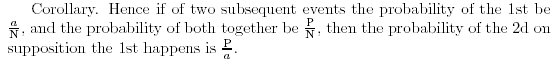Search

Useful Lists

Partners

Online Manuals

Implement Bayesian inference using PHP, Part 1
By Paul Meagher - 2004-04-21 Page:  1 2 3 4 5 6 7 8 9 10 11

## Frequency versus probability format

The `getConditionalProbability` function you've developed operates on counts and frequencies rather than on probabilities. In reading the literature on Bayesian reasoning, you will notice that the enumeration method for computing P(A | B) is only briefly discussed. Most authors quickly move onto describing how P(A | B) can be formulated using terms denoting probability values rather than frequency counts. For example, you can recast the formula for computing P(A | B) using such probability terms as:

P(A | B) = P(A & B) / P(B)

The advantage of recasting the formula using terms denoting probabilities instead of frequency counts arises because in practice, you often don't have access to a data set we can use to derive conditional probability estimates through an enumeration of cases method. Instead, you often have access to higher-level summary information from past studies in the form of percentages and probabilities. With the available information, the challenge then becomes finding a way to use these probability estimates instead to compute the conditional probabilities you are interested in. Recasting the conditional probability formula in terms of probabilities allows you to make inferences based on related probability information that is more readily accessible.

The enumeration method might still be regarded as the most basic and intuitive method for computing a conditional probability. In Thomas Bayes' "Essay on the Doctrine of Chances," he uses enumeration to arrive at the conclusion that P( 2nd Event = b | 1st Event = a ) is equal to [P / N] / [ a / N], which is equal to P / a, which one can also denote as {a & b} / {a}:

Figure 1. Graphical representation of relationsAnother reason why it is important to be aware of frequency versus probability format issues is because it has been demonstrated by Gerd Gigerenzer (and others) that people are better at reasoning in accordance with prescriptive Bayesian rules of inference when background information is presented in terms of frequencies of cases (1 in 10 cases) rather than probabilities (10 percent probability). A practical application of this research is that medical students are now being taught to communicate risk information in terms of frequencies of cases instead of probabilities, making it easier for patients to make better informed judgements about what actions are warranted given the test results.

View Implement Bayesian inference using PHP, Part 1 Discussion

Page:  1 2 3 4 5 6 7 8 9 10 11 Next Page: Joint probability